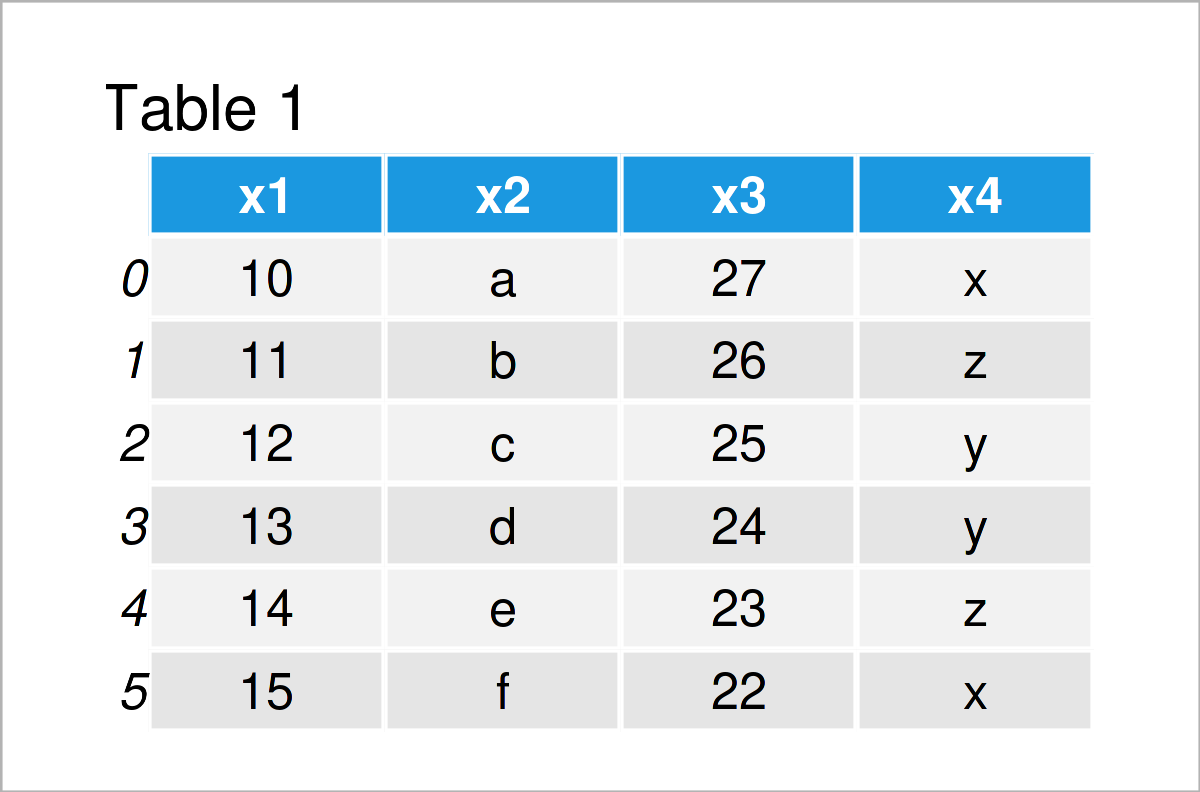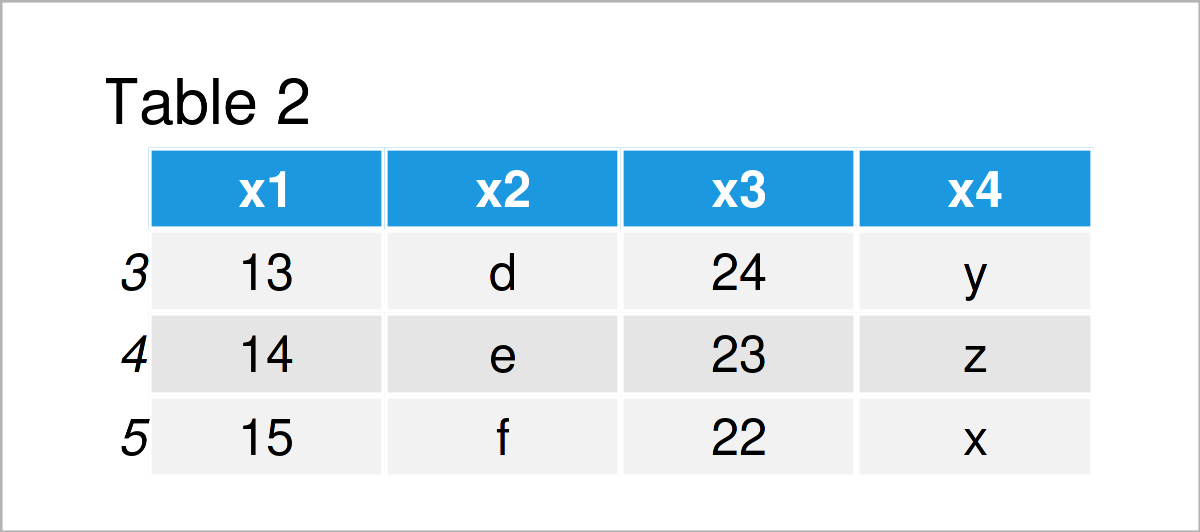# Drop First & Last N Rows from pandas DataFrame in Python (2 Examples)

In this Python article you’ll learn how to delete the first and last N rows from a pandas DataFrame.

The post will contain two examples for the deletion of the first and last N rows from a pandas DataFrame. To be more precise, the post will consist of the following information:

So now the part you have been waiting for – the examples!

## Example Data & Software Libraries

In order to use the functions of the pandas library, we first need to load pandas to Python:

`import pandas as pd # Import pandas library`

In addition, have a look at the following example data:

```data = pd.DataFrame({'x1':range(10, 16), # Create pandas DataFrame 'x2':['a', 'b', 'c', 'd', 'e', 'f'], 'x3':range(27, 21, - 1), 'x4':['x', 'z', 'y', 'y', 'z', 'x']}) print(data) # Print pandas DataFrame```Table 1 shows that our example DataFrame has six rows and four columns.

## Example 1: Drop First N Rows from pandas DataFrame in Python

In this example, I’ll explain how to delete the first N rows from a pandas DataFrame.

For this, we can use the iloc indexer as shown below:

```data_drop_first = data.iloc[3:] # Using iloc indexer print(data_drop_first) # Print updated DataFrame```After executing the previous Python code the new pandas DataFrame object shown in Table 2 has been created. As you can see, the first three rows of our input data set have been removed.

## Example 2: Drop Last N Rows from pandas DataFrame in Python

Example 2 demonstrates how to drop the last N rows from a pandas DataFrame.

To do this, we can use the iloc indexer once again:

```data_drop_last = data.iloc[:3] # Using iloc indexer print(data_drop_last) # Print updated DataFrame```As shown in Table 3, we have created another pandas DataFrame where the last three rows have been deleted.

## Video & Further Resources

I have recently released a video on my YouTube channel, which shows the topics of this tutorial. You can find the video below.

In addition, you might have a look at the other tutorials on my website. I have released several articles on topics such as indices, extracting data, counting, and descriptive statistics:

You have learned in this article how to remove the head and tail from a pandas DataFrame in the Python programming language. Don’t hesitate to let me know in the comments section, in case you have any additional questions and/or comments.

Subscribe to the Statistics Globe Newsletter